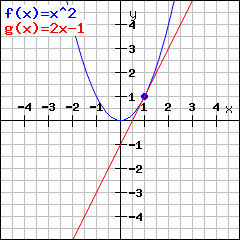# Derivation calculatorEnter the function that shall be differentiated. Hint: Enteras 3*x^2 ,as 3/5 andas (x+1)/(x-2x^4)

## What is the derivation?

The derivation of a function at x says what slope the graph of the function has at x, i.e. what slope a tangent line in the point (x|f(x)) has.Example: The normal parabolahas the tangent linein (1|1), i.e. slope. The derivation of the normal parabola atthus equals.

## What's the difference between the derivation and the derivation function?

The derivation function f'(x) of f(x) is a function giving the slope at x for evey given x. This means: To find out what the slope of f at x is, you just have to enter x into the derivation function .

## And how to calculate a derivation?

Before finding the differentiation rules, one has to calculate the difference quotient for every point separately. By using differentiation rules, things become simpler: First, you calculate the derivation of power functions.. This is simply. Further rules make it possible to calculate the derivation of an arbitrary polynomial function, cause it is just the sum of products of power functions and numbers. So you need those rules:
• the factor rule:• and the sum rule: The derivation ofequalsFor more complicatet functions, there are further derivation rules needed:
• the product rule: The derivation ofequals• the quotient rule: The derivation ofequals• the chain rule: The derivation ofequals## Why to find roots of the derivation?

The roots of derivation are important points of the graph. At maximum or minimum turning points, the first derivation equals zero. (Trouble spot: But just because the first derivation is zero, a point does not have to be a turning point! Check out change of signs for further information. ) At an inflection point the second derivation is zero. So you can find out much about your function by putting the derivation equal to zero and solving the equation.